# Temperature + subtraction - math problems

#### Number of problems found: 56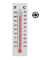Please help, I'm lost! I'm calculating the difference between two Fahrenheit degrees 55F - 37F = 18 F Now if I converted the degrees to Celsius before subtracting then we get 12.78C - 2.78 =10C How is that possible when 18F does not equal 10C. Could yo pl
• The temperature 19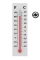The temperature during the day in a cold country is 9 degrees celsius. At night the temperature falls by 13 degrees celsius. What is the temperature at night?
• When Joe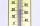When Joe went to work at 6 a. M. , the outside temperature was 3 degrees Celsius. During the day, the Weather worsened and by 3 p. M. the temperature dropped 5 degrees. What was the temperature at 5 p. M. ?
• An experiment 2The temperature of a substance in an experiment changes by -8.7°F from 10 AM to 1 PM At 5 PM, the temperature is 33.5°F. It is 2/3 of what the temperature was at 1 PM. What was the temperature at 10 AM of the substance?
• Change per hour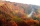On Sunday, the temperature was 63 degrees at 2 pm. The temperature was then 72 degrees at 5 pm. What is the rate of change per hour?
• The temperature 16The temperature at 6 am was 21 degrees Fahrenheit by noon, and the temperature dropped 32 degrees Fahrenheit. What was the temperature at noon?
• The temp rangeWhat is the range in temperatures if the high temperatures is 67 degrees Celsius and the low temperature is -25 degrees Celsius?
• The temperature 14The temperature is 12°C lower than 4 hours ago. a. What is the average temperature change per hour? b. Write an integer equation for the situation.
• The level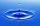The temperature of the liquid in a thermometer is 24.32°C lower than the boiling point of water. What is the thermometer reading? Show your answer by drawing and shading the thermometer.
• Temperature up and dowmAt 6 AM, the temperature is 19.4°C. Between 6 AM and noon, the temperature rises by 3.8°C. Between noon and 6 PM, the temperature falls 2.5 °C. What is the temperature at 6 PM?
• Lower temperatureThe level of temperature of the liquid in a thermometer is 24.32°C lower than the boiling point of water. What is the thermometer reading? Show your answer by drawing and shading the thermometer.
• Now isNow is 5°C morning. What will the temperature be if i. It rises by 12°C ii. It falls by 15°C
• The temperature 11The temperature on Sunday was 4°C. The temperature dropped 8°C on Monday and twice as much on Tuesday. What is the temperature on Tuesday?
• RoxvilleThe temperature in Roxville decreased 15 degree in five hours. What was the average decrease in temperature per hour? Write you answer as an integer.
• At noon 2At noon, the temperature was 4 degrees fahrenheit. At 6pm, it was 10 degrees colder. What was the temperature at 6pm?
• If the 3If the temperature of a city drops 2° everyday and increases by 3° on every 5 days, how long does it take to drop to -15° from temperature +5°?
• Ground levelThe temperature at ground level in Helsinki is 20°C lower than in London. The temperature in a basement flat in Helsinki is 5°C below the temperature at ground level. If it is -29°c in the basement, what is the temperature in London?
• At midmorningThe temperature at midmorning was 31°C. Early in the evening, the temperature dropped by 5°C. What was the resulting temperature?
• WeatherWeather. At 5:00 am, the temperature was 28°C, and at noontime, it rose by 9°C. By 4:00 pm, it dropped 3°C, and by nighttime, it was 4°C less. What is the room temperature this time?
• Temperature rise and fallOn Friday, the temperature was 82°F. The temperature changed by –2°F on Saturday, and then it changed by 5°F on Sunday. What was the temperature on Sunday? How did the temperature change? Note we consider the mathematical problem formulated in this way to

Do you have an exciting math question or word problem that you can't solve? Ask a question or post a math problem, and we can try to solve it.

We will send a solution to your e-mail address. Solved examples are also published here. Please enter the e-mail correctly and check whether you don't have a full mailbox.

Temperature - math problems. Subtraction Problems.